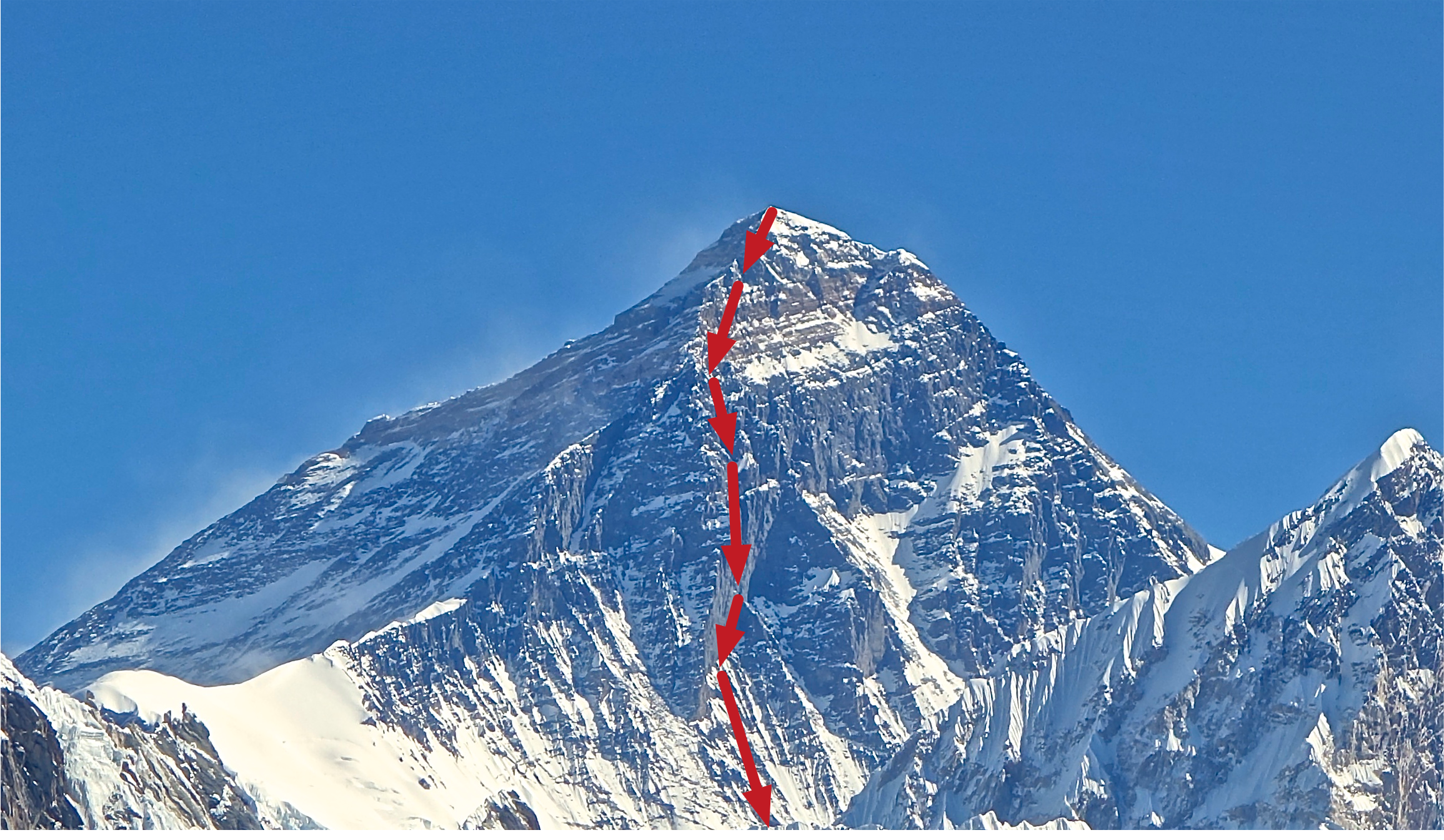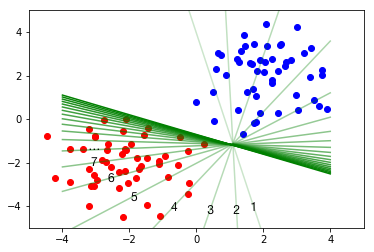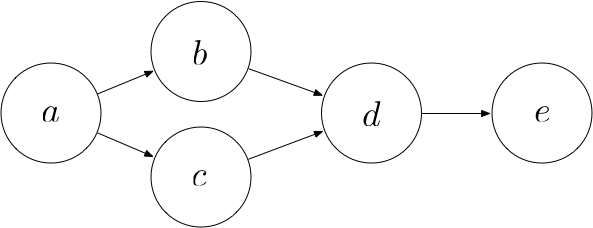This is part 4 of a series of tutorials, in which we develop the mathematical and algorithmic underpinnings of deep neural networks from scratch and implement our own neural network library in Python, mimicing the TensorFlow API. Start with the first part: I: Computational Graphs.

Generally, if we want to find the minimum of a function, we set the derivative to zero and solve for the parameters. It turns out, however, that it is impossible to obtain a closed-form solution for $W$ and $b$. Instead, we iteratively search for a minimum using a method called gradient descent.

As a visual analogy, imagine yourself standing on a mountain and trying to find the way down. At every step, you walk into the steepest direction, since this direction is the most promising to lead you towards the bottom.If taking steep steps seems a little dangerous to you, imagine that you are a mountain goat (which are amazing rock climbers).

Gradient descent operates in a similar way when trying to find the minimum of a function: It starts at a random location in parameter space and then iteratively reduces the error $J$ until it reaches a local minimum. At each step of the iteration, it determines the direction of steepest descent and takes a step along that direction. This process is depicted for the 1-dimensional case in the following image.As you might remember, the direction of steepest ascent of a function at a certain point is given by the gradient at that point. Therefore, the direction of steepest descent is given by the negative of the gradient. So now we have a rough idea how to minimize $J$:

1. Start with random values for $W$ and $b$
2. Compute the gradients of $J$ with respect to $W$ and $b$
3. Take a small step along the direction of the negative gradient
4. Go back to 2

Let’s implement an operation that minimizes the value of a node using gradient descent. We require the user to specify the magnitude of the step along the gradient as a parameter called learning_rate.

The following image depicts an example iteration of gradient descent. We start out with a random separating line (marked as 1), take a step, arrive at a slightly better line (marked as 2), take another step, and another step, and so on until we arrive at a good separating line.# Backpropagation

In our implementation of gradient descent, we have used a function compute_gradient(loss) that computes the gradient of a $loss$ operation in our computational graph with respect to the output of every other node $n$ (i.e. the direction of change for $n$ along which the loss increases the most). We now need to figure out how to compute gradients.

Consider the following computational graph:By the chain rule, we have

$$\frac{\partial e}{\partial a} = \frac{\partial e}{\partial b} \cdot \frac{\partial b}{\partial a} = \frac{\partial e}{\partial c} \cdot \frac{\partial c}{\partial b} \cdot \frac{\partial b}{\partial a} = \frac{\partial e}{\partial d} \cdot \frac{\partial d}{\partial c} \cdot \frac{\partial c}{\partial b} \cdot \frac{\partial b}{\partial a}$$

As we can see, in order to compute the gradient of $e$ with respect to $a$, we can start at $e$ an go backwards towards $a$, computing the gradient of every node’s output with respect to its input along the way until we reach $a$. Then, we multiply them all together.

Now consider the following scenario:In this case, $a$ contributes to $e$ along two paths: The path $a$, $b$, $d$, $e$ and the path $a$, $c$, $d$, $e$. Hence, the total derivative of $e$ with respect to $a$ is given by:

$$\frac{\partial e}{\partial a} = \frac{\partial e}{\partial d} \cdot \frac{\partial d}{\partial a} = \frac{\partial e}{\partial d} \cdot \left( \frac{\partial d}{\partial b} \cdot \frac{\partial b}{\partial a} + \frac{\partial d}{\partial c} \cdot \frac{\partial c}{\partial a} \right) = \frac{\partial e}{\partial d} \cdot \frac{\partial d}{\partial b} \cdot \frac{\partial b}{\partial a} + \frac{\partial e}{\partial d} \cdot \frac{\partial d}{\partial c} \cdot \frac{\partial c}{\partial a}$$

This gives us an intuition for the general algorithm that computes the gradient of the loss with respect to another node: We perform a backwards breadth-first search starting from the loss node. At each node $n$ that we visit, we compute the gradient of the loss with respect do $n$’s output by doing the following for each of $n$’s consumers $c$:

• retrieve the gradient $G$ of the loss with respect to the output of $c$
• multiply $G$ by the gradient of $c$’s output with respect to $n$’s output

And then we sum those gradients over all consumers.

As a prerequisite to implementing backpropagation, we need to specify a function for each operation that computes the gradients with respect to the inputs of that operation, given the gradients with respect to the output. Let’s define a decorator @RegisterGradient(operation_name) for this purpose:

Now assume that our _gradient_registry dictionary is already filled with gradient computation functions for all of our operations. We can now implement backpropagation:

For each of our operations, we now need to define a function that turns a gradient of the loss with respect to the operation’s output into a list of gradients of the loss with respect to each of the operation’s inputs. Computing a gradient with respect to a matrix can be somewhat tedious. Therefore, the details have been omitted and I just present the results. You may skip this section and still understand the overall picture.

If you want to comprehend how to arrive at the results, the general approach is as follows:

• Find the partial derivative of each output value with respect to each input value (this can be a tensor of a rank greater than 2, i.e. neither scalar nor vector nor matrix, involving a lot of summations)
• Compute the gradient of the loss with respect to the node’s inputs given a gradient with respect to the node’s output by applying the chain rule. This is now a tensor of the same shape as the input tensor, so if the input is a matrix, the result is also a matrix
• Rewrite this result as a sequence of matrix operations in order to compute it efficiently. This step can be somewhat tricky.

### Gradient for negative

Given a gradient $G$ with respect to $-x$, the gradient with respect to $x$ is given by $-G$.

### Gradient for log

Given a gradient $G$ with respect to $log(x)$, the gradient with respect to $x$ is given by $\frac{G}{x}$.

### Gradient for sigmoid

Given a gradient $G$ with respect to $\sigma(a)$, the gradient with respect to $a$ is given by $G \cdot \sigma(a) \cdot \sigma(1-a)$.

### Gradient for multiply

Given a gradient $G$ with respect to $A \odot B$, the gradient with respect to $A$ is given by $G \odot B$ and the gradient with respect to $B$ is given by $G \odot A$.

### Gradient for matmul

Given a gradient $G$ with respect to $AB$, the gradient with respect to $A$ is given by $GB^T$ and the gradient with respect to $B$ is given by $A^TG$.

### Gradient for add

Given a gradient $G$ with respect to $a + b$, the gradient with respect to $a$ is given by $G$ and the gradient with respect to $b$ is also given by $G$, provided that $a$ and $b$ are of the same shape. If $a$ and $b$ are of different shapes, e.g. one matrix $a$ with 100 rows and one row vector $b$, we assume that $b$ is added to each row of $a$. In this case, the gradient computation is a little more involved, but I will not spell out the details here.

### Gradient for reduce_sum

Given a gradient $G$ with respect to the output of reduce_sum, the gradient with respect to the input $A$ is given by repeating $G$ along the specified axis.

## Example

Let’s now test our implementation to determine the optimal weights for our perceptron.

Notice that we started out with a rather high loss and incrementally reduced it. Let’s plot the final line to check that it is a good separator:

If you have any questions, feel free to leave a comment. Otherwise, continue with the next part: V: Multi-Layer Perceptrons